## Question of the Week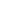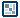Isometric
Hit Testing
by Danko Kozar : 3 December 2004

This is the second tutorial of the "isometric game" series. The first one, in case you haven't read it, involves isometric transformations. Anyway, in this tutorial I'll explain hit testing in the 3d isometric perspective.

So what is this all about? What's wrong with the good old hitTest function?
Well, this: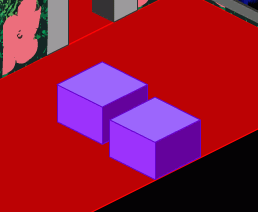[ two objects that are NOT overlapping ]

Look at the figure above. You can see two boxes that are lying on the floor and not touching each other.

But, since Flash's hitTest function works only in plain 2d, the result of testing a hit between these two objects would be true.

Now, what can we do to avoid that?

As I said in the previous tutorial, the way out of this is to do all our calculations in 3d isometric space, and then transform it into plain 2d Flash graphics.

Back to 2d
For the sake of simplicity, the next example will be using a "top" projection, and will be dealing with 2 dimensions only. When the logic is understood, the third dimension is added in a minute, and we have a perfectly functional 3d hit test.

Introducing new function called hitTest2:

function hitTest2(obj1, obj2) {
if ((Math.abs((obj1.x+obj1.a/2)-(obj2.x+obj2.a/2))<(obj1.a+obj2.a)/2) and (Math.abs((obj1.y+obj1.b/2)-(obj2.y+obj2.b/2))<(obj1.b+obj2.b)/2)) {
return (true);
}
}

This function calculates the relationship between two objects.

The parameters are 2 objects; it returns true if they are overlapping, false if not.

The x, y, a and b are the properties of each object. They represent x and y coordinate and objects width and height.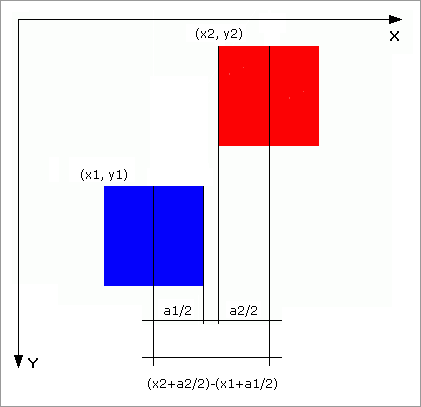[ checking the (x axis) distance between centers ]

The math behind rectangular hit testing is pretty simple. Basically, it checks if the distance between centers of the objects is less then sum of the halves of their widths. If it is - they overlap on that axis.

The figure above shows checking that distance for x direction (x axis). In this particular case, the objects don't overlap on an x axis.

The function does the same thing for x and y direction (and z direction, later in 3d).[ overlapping in 2d ]

If objects overlap on x and y axis at the same time - they overlap in general.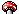Note
 As you see, I use the upper left edge coordinates of the object to represent the object's position. Since hitTest2 function deals with distances between the object centres, it's handy to use object's center coordinates to represent the object position. I could have done it that way too.

Let's try it:

[ click the screen and use arrow keys to move the red square ]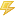Note
 The drawback of this function is that it assumes all objects are rectangular. A ball can be drawn as a ball, but for a hit testing purposes - it's a cube. There's nothing wrong with it - all filmation isometric games use rectangular objects.

Onwards to the next page!